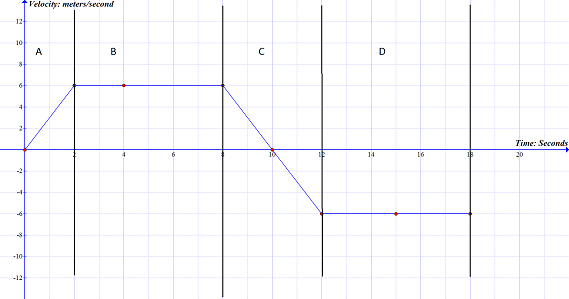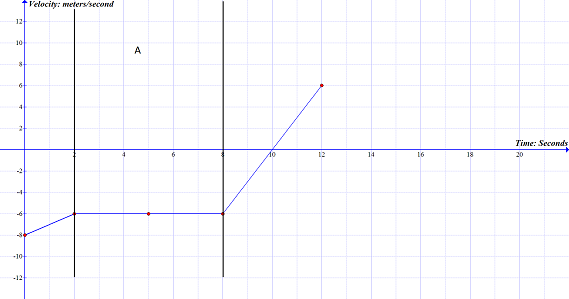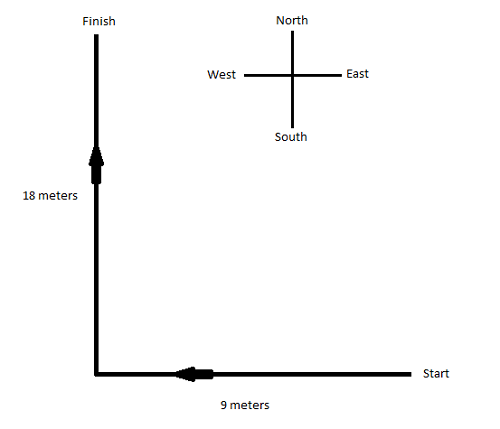# Dimensions of Motion in Physics Chapter Exam

Exam Instructions:

Choose your answers to the questions and click 'Next' to see the next set of questions. You can skip questions if you would like and come back to them later with the yellow "Go To First Skipped Question" button. When you have completed the practice exam, a green submit button will appear. Click it to see your results. Good luck!

### Page 2

#### Question 7 7. Using the graph, calculate the displacement for the section labeled B.#### Question 8 8. Looking at this graph, what assumption can you make about the displacement of the object over the time period indicated by the letter A?### Page 3

#### Question 13 13. Using the graph, calculate the displacement for the section labeled A.### Page 6

#### Question 26 26. Calculate the distance travelled by the object in the diagram.#### Question 29 29. Using the graph, calculate the displacement for the section labeled D.#### Question 30 30. Calculate the displacement for some object in the diagram that starts at Start and ends at Finish.#### Dimensions of Motion in Physics Chapter Exam Instructions

Choose your answers to the questions and click 'Next' to see the next set of questions. You can skip questions if you would like and come back to them later with the yellow "Go To First Skipped Question" button. When you have completed the practice exam, a green submit button will appear. Click it to see your results. Good luck!

Support